# Trávník's smooth self-referential formula - The limit and a role of epsilon

## Limit

The formula includes following function with a limit:The limit represents ideal of Bézier curves. But practically just using sufficiently small number ε is enough. There is no need to try compute the limit for displaying the formula. Let me explain this in pictures on area of the formula for:
2.05 <= x < 3.75
35.45 <= y < 36.15

Rendering with ε=0.5: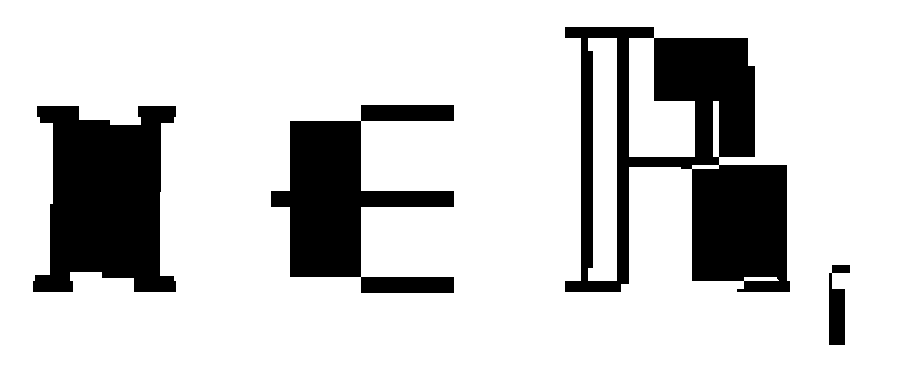Rendering with ε=0.1: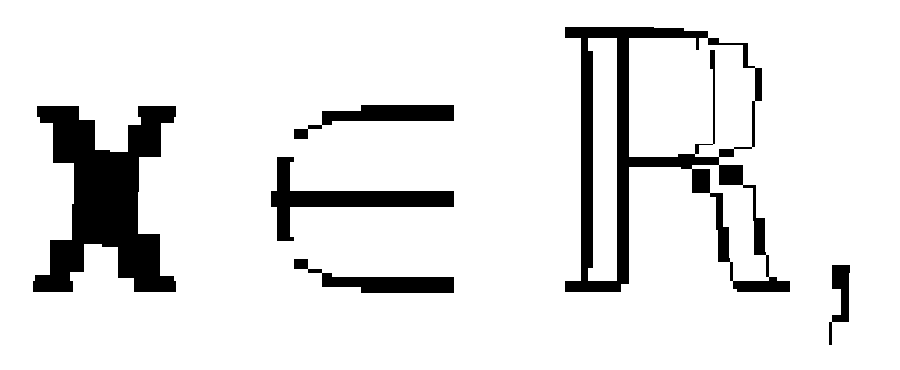Rendering with ε=0.01:Rendering with ε=0.00001: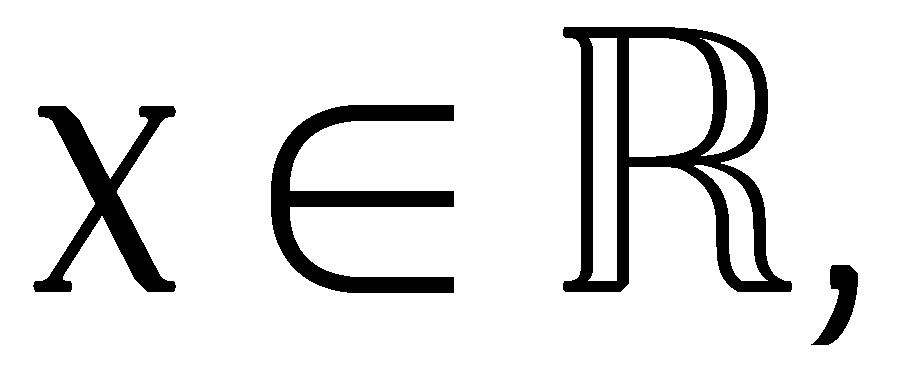Rendering with ε=0.00000001:The ε just needs to be significantly smaller than pixel width. In above image pixel width is 0.00188. It is also useful to have it smaller than resolution of smallest detail which could have impact on aliasing.

I am aware that limit is not defined on the edges of shapes because of their expected discontinuity. But I'm happy that is it defined everywhere else.

In some sense the limit achieves nothing. What would happen if the limit would be removed along with ε and corresponding branching in Wc function that terminates recursion with π value when bounding box diagonal is smaller than ε? The function would be well defined everywhere except on the edges of shapes. On edges the recursive definition would not terminate making it not well defined there. That is pretty similar to what happens when this limit is present. But I still like the limit to express intent that you can get close with small enough ε and that you have a prescribed way how to terminate this unlimited recursion. There are different ways to terminate the recursion. Here is another different way:

There is one modification of Wc function in d < ε branch:

```Wc(d, x, y, x1, y1, x2, y2, x3, y3, x4, y4, ε)
= π if d < ε ∧ ((x4−x1)⋅(y−y1)−(y4−y1)⋅(x−x1)) > 0
= −π if d < ε ∧ ((x4−x1)⋅(y−y1)−(y4−y1)⋅(x−x1)) ≤ 0
= Wa(x, y, x1, y1, (x1+x2)/2, (y1+y2)/2, (x2+x3+x1+x2)/4, (y2+y3+y1+y2)/4, (x1+3⋅x2+3⋅x3+x4)/8, (y4+3⋅y3+3⋅y2+y1)/8, ε)
+Wa(x, y, (x4+3⋅x3+3⋅x2+x1)/8, (y4+3⋅y3+3⋅y2+y1)/8, (x4+2⋅x3+x2)/4, (y4+2⋅y3+y2)/4, (x3+x4)/2, (y3+y4)/2, x4, y4, ε),  else
```

This results in line x1,y1 to x4,y4 approximation where rendered pixel coordinates are closer to curve than ε.

Class SelfFormulaFastEpsSmoother in sources which implements this modified variant. See how it converges with various ε values. It looks nicer:

Rendering with ε=0.5: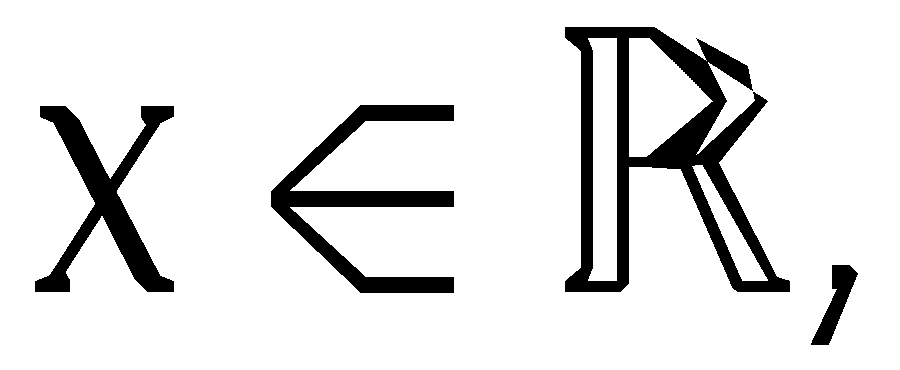Rendering with ε=0.1: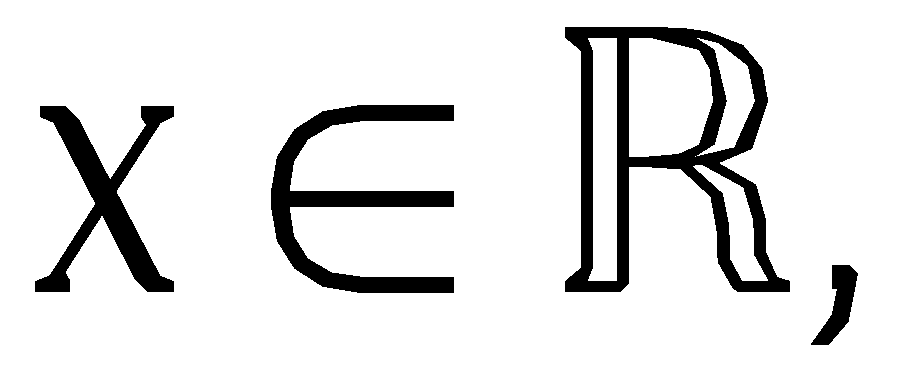Rendering with ε=0.01:Rendering with ε=0.00001: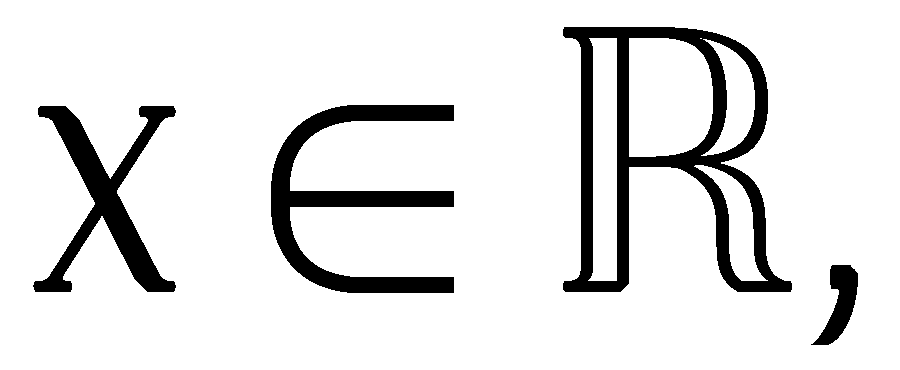Rendering with ε=0.00000001:So it is much smoother, but I did not want to complicate the formula so it contains the concise variant of Wc function.

Back to index - Jakub Trávník's resources.